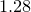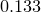# 7.4 Foreach datum loops

Foreach datum loops are similar to foreach loops in that they run a script block once for each item in a list. In this case, however, the list in question is the list of data points in a data file, samples of a function, or values in a vector. The syntax of the foreach datum command is similar to that of the commands met in the previous chapter for acting on data files: the standard modifiers every, index, select and using can be used to select which columns of the data file, and which subset of the datapoints, should be used:

```foreach datum i,j,name in "data.dat" using 1:2:"%s"%(\$3)
<code>

foreach datum x,y,z in sin(x):cos(x)
<code>

foreach datum a,b in vector_a:vector_b
<code>
```

The foreach datum command is followed by a comma-separated list of the variable(s) which are to be read from the input data on each iteration of the loop. The using modifier specifies the columns or rows of data which are to be used to set the values of each variable. In the first example above, the third variable, name, is set as a string, indicating that it will be set to equal whatever string of text is found in the third column of the data file.

Example: Calculating the mean and standard deviation of data

The following Pyxplot script calculates the mean and standard deviation of a set of data points using the foreach datum command:
N_data = 0
sum_x = 0
sum_x2 = 0

foreach datum x in ’–’
{
N_data ++
sum_x += x
sum_x2 += x**2
}
1.3
1.2
1.5
1.1
1.3
END

mean = sum_x / N_data
SD = sqrt(sum_x2 / N_data - mean**2)

print "Mean = %s"%mean
print "SD = %s"%SD
For the data supplied, a mean ofand a standard deviation ofare returned.